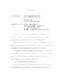## Critical thresholds in Eulerian dynamics##### Files
umi-umd-4627.pdf(363.08 KB)
In this thesis, we study the critical regularity phenomena in Eulerian dynamics, $u_t+u\cdot \nabla u=F(u,D u,\cdots),$ here $F$ represents a general force acting on the flow and by regularity we seek to obtain a large set of sub-critical initial data. We analyze three prototype models, ranging from the one-dimensional Euler-Poisson equations to two-dimensional system of Burgers equations to three-dimensional, four-dimensional and even higher-dimensional restricted Euler systems. We begin with the one-dimensional Euler-Poisson equations, where $F$ is the Poisson forcing term together with the usual $\gamma$-law pressure. We prove that global regularity of the Euler-Poisson equations with $\gamma\geq 1$ depends on whether or not the initial configuration crosses an intrinsic critical threshold. Next, we discuss multi-dimensional examples. The first multi-dimensional example that we focus our attention on is the two dimensional pressureless flow, where $F=\epsilon \Delta u$. Our analysis shows that there is a uniform \textsl{BV} bound of the solutions $u^{\epsilon}$. Moreover, if the initial velocity gradient $\nabla u_0$ does not have negative eigenvalues, then its vanishing viscosity limit is the smooth solution of the corresponding equations of the inviscid fluid flow. The second multi-dimensional example we discuss here is the restricted Euler dynamics, where $\nabla F= \displaystyle\frac{1}{n} \mathrm {tr} (\nabla u)^2I_{n\times n}$\,. Our analysis shows that for the three-dimensional case, the finite-time breakdown of the restricted Euler system is generic, and for the four-dimensional case, there is a surprising global existence for sub-critical initial data. Further analysis extends the above result to the general $n$-dimensional ($n>4$) restricted Euler system.